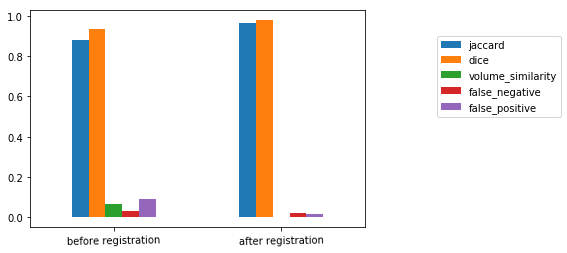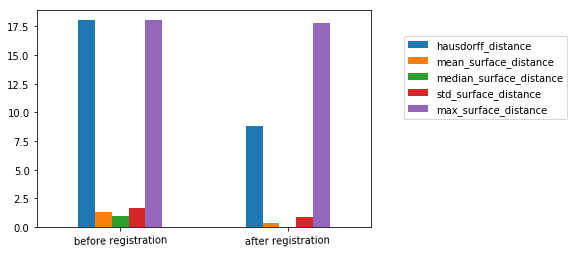Summary:

1. SimpleITK provides two flavors of non-rigid registration:
• Free Form Deformation, BSpline based, and Demons using the ITKv4 registration framework.
• A set of Demons filters that are independent of the registration framework (DemonsRegistrationFilter, DiffeomorphicDemonsRegistrationFilter, FastSymmetricForcesDemonsRegistrationFilter, SymmetricForcesDemonsRegistrationFilter).
2. Registration evaluation:
• Registration accuracy, the quantity of interest is the Target Registration Error (TRE).
• TRE is spatially variant.
• Surrogate metrics for evaluating registration accuracy such as segmentation overlaps are relevant, but are potentially deficient.
• Registration time.
• Acceptable values for TRE and runtime are context dependent.
In :
import SimpleITK as sitk
import registration_gui as rgui
import utilities

from ipywidgets import interact, fixed

%matplotlib inline
import matplotlib.pyplot as plt

import numpy as np

In this notebook we will use the Point-validated Pixel-based Breathing Thorax Model (POPI). This is a 4D (3D+time) thoracic-abdominal CT (10 CTs representing the respiratory cycle) with masks segmenting each of the CTs to air/body/lung, and a set of corresponding landmarks localized in each of the CT volumes.

The registration problem we deal with is non-rigid alignment of the lungs throughout the respiratory cycle. This information is relevant for radiation therapy planning and execution.

The POPI model is provided by the Léon Bérard Cancer Center & CREATIS Laboratory, Lyon, France. The relevant publication is:

J. Vandemeulebroucke, D. Sarrut, P. Clarysse, "The POPI-model, a point-validated pixel-based breathing thorax model", Proc. XVth International Conference on the Use of Computers in Radiation Therapy (ICCR), Toronto, Canada, 2007.

Additional 4D CT data sets with reference points are available from the CREATIS Laboratory here.

In :
images = []
points = []
image_indexes = [0,7]
for i in image_indexes:
image_file_name = 'POPI/meta/{0}0-P.mhd'.format(i)
points_file_name = 'POPI/landmarks/{0}0-Landmarks.pts'.format(i)

interact(rgui.display_coronal_with_overlay, temporal_slice=(0,len(images)-1),
coronal_slice = (0, images.GetSize()-1),
label=fixed(utilities.popi_lung_label), window_min = fixed(-1024), window_max=fixed(976));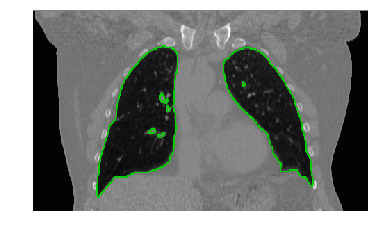Free Form Deformation¶

Define a BSplineTransform using a sparse set of grid points overlaid onto the fixed image's domain to deform it.

For the current registration task we are fortunate in that we have a unique setting. The images are of the same patient during respiration so we can initialize the registration using the identity transform. Additionally, we have masks demarcating the lungs.

We use the registration framework taking advantage of its ability to use masks that limit the similarity metric estimation to points lying inside our region of interest, the lungs.

In :
fixed_index = 0
moving_index = 1

fixed_image = images[fixed_index]
fixed_points = points[fixed_index]

moving_image = images[moving_index]
moving_points = points[moving_index]
In :
# Define a simple callback which allows us to monitor registration progress.
def iteration_callback(filter):
print('\r{0:.2f}'.format(filter.GetMetricValue()), end='')

registration_method = sitk.ImageRegistrationMethod()

# Determine the number of BSpline control points using the physical
# spacing we want for the finest resolution control grid.
grid_physical_spacing = [50.0, 50.0, 50.0] # A control point every 50mm
image_physical_size = [size*spacing for size,spacing in zip(fixed_image.GetSize(), fixed_image.GetSpacing())]
mesh_size = [int(image_size/grid_spacing + 0.5) \
for image_size,grid_spacing in zip(image_physical_size,grid_physical_spacing)]
# The starting mesh size will be 1/4 of the original, it will be refined by
# the multi-resolution framework.
mesh_size = [int(sz/4 + 0.5) for sz in mesh_size]

initial_transform = sitk.BSplineTransformInitializer(image1 = fixed_image,
transformDomainMeshSize = mesh_size, order=3)
# Instead of the standard SetInitialTransform we use the BSpline specific method which also
# accepts the scaleFactors parameter to refine the BSpline mesh. In this case we start with
# the given mesh_size at the highest pyramid level then we double it in the next lower level and
# in the full resolution image we use a mesh that is four times the original size.
registration_method.SetInitialTransformAsBSpline(initial_transform,
inPlace=False,
scaleFactors=[1,2,4])

registration_method.SetMetricAsMeanSquares()
registration_method.SetMetricSamplingStrategy(registration_method.RANDOM)
registration_method.SetMetricSamplingPercentage(0.01)

registration_method.SetShrinkFactorsPerLevel(shrinkFactors = [4,2,1])
registration_method.SetSmoothingSigmasPerLevel(smoothingSigmas=[2,1,0])
registration_method.SmoothingSigmasAreSpecifiedInPhysicalUnitsOn()

registration_method.SetInterpolator(sitk.sitkLinear)
registration_method.SetOptimizerAsLBFGS2(solutionAccuracy=1e-2, numberOfIterations=100, deltaConvergenceTolerance=0.01)

final_transformation = registration_method.Execute(fixed_image, moving_image)
print('\nOptimizer\'s stopping condition, {0}'.format(registration_method.GetOptimizerStopConditionDescription()))
3812.56
Optimizer's stopping condition, Reached maximum number of iterations

Qualitative evaluation via segmentation transfer¶

Transfer the segmentation from the moving image to the fixed image before and after registration and visually evaluate overlap.

In :
fixed_image,
final_transformation,
sitk.sitkNearestNeighbor,
0.0,

interact(rgui.display_coronal_with_overlay, temporal_slice=(0,1),
coronal_slice = (0, fixed_image.GetSize()-1),
label=fixed(1), window_min = fixed(-1024), window_max=fixed(976));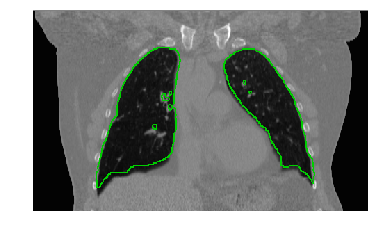Quantitative evaluation¶

The most appropriate evaluation is based on analysis of Target Registration Errors(TRE), which is defined as follows:

Given the transformation $T_f^m$ and corresponding points in the two coordinate systems, $^fp,^mp$, points which were not used in the registration process, TRE is defined as $\|T_f^m(^fp) - ^mp\|$.

We start by looking at some descriptive statistics of TRE:

In :
initial_TRE = utilities.target_registration_errors(sitk.Transform(), fixed_points, moving_points)
final_TRE = utilities.target_registration_errors(final_transformation, fixed_points, moving_points)

print('Initial alignment errors in millimeters, mean(std): {:.2f}({:.2f}), max: {:.2f}'.format(np.mean(initial_TRE),
np.std(initial_TRE),
np.max(initial_TRE)))
print('Final alignment errors in millimeters, mean(std): {:.2f}({:.2f}), max: {:.2f}'.format(np.mean(final_TRE),
np.std(final_TRE),
np.max(final_TRE)))
Initial alignment errors in millimeters, mean(std): 5.07(2.67), max: 14.02
Final alignment errors in millimeters, mean(std): 1.60(0.89), max: 4.41

The above descriptive statistics do not convey the whole picture, we should also look at the TRE distributions before and after registration.

In :
plt.hist(initial_TRE, bins=20, alpha=0.5, label='before registration', color='blue')
plt.hist(final_TRE, bins=20, alpha=0.5, label='after registration', color='green')
plt.legend()
plt.title('TRE histogram');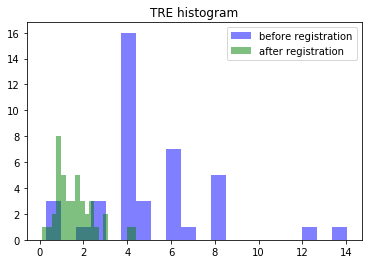Finally, we should also take into account the fact that TRE is spatially variant (think center of rotation). We therefore should also explore the distribution of errors as a function of the point location.

In :
initial_errors = utilities.target_registration_errors(sitk.Transform(), fixed_points, moving_points, display_errors = True)
utilities.target_registration_errors(final_transformation, fixed_points, moving_points,
min_err=min(initial_errors), max_err=max(initial_errors), display_errors = True);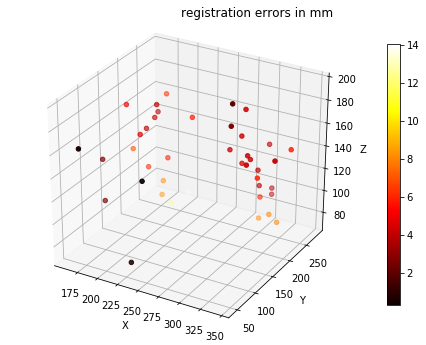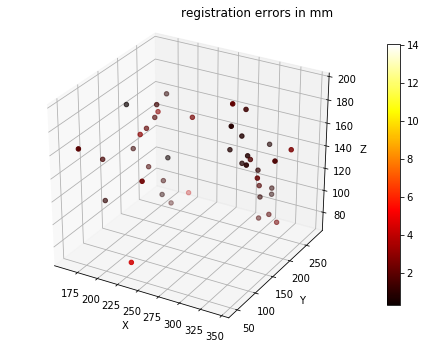Deciding whether a registration algorithm is appropriate for a specific problem is context dependent and is defined by the clinical/research needs both in terms of accuracy and computational complexity.

Demons Based Registration¶

SimpleITK includes a number of filters from the Demons registration family (originally introduced by J. P. Thirion):

1. DemonsRegistrationFilter.
2. DiffeomorphicDemonsRegistrationFilter.
3. FastSymmetricForcesDemonsRegistrationFilter.
4. SymmetricForcesDemonsRegistrationFilter.

These are appropriate for mono-modal registration. As these filters are independent of the ImageRegistrationMethod we do not have access to the multiscale framework. Luckily it is easy to implement our own multiscale framework in SimpleITK, which is what we do in the next cell.

In :
def smooth_and_resample(image, shrink_factor, smoothing_sigma):
"""
Args:
image: The image we want to resample.
shrink_factor: A number greater than one, such that the new image's size is original_size/shrink_factor.
smoothing_sigma: Sigma for Gaussian smoothing, this is in physical (image spacing) units, not pixels.
Return:
Image which is a result of smoothing the input and then resampling it using the given sigma and shrink factor.
"""
smoothed_image = sitk.SmoothingRecursiveGaussian(image, smoothing_sigma)

original_spacing = image.GetSpacing()
original_size = image.GetSize()
new_size = [int(sz/float(shrink_factor) + 0.5) for sz in original_size]
new_spacing = [((original_sz-1)*original_spc)/(new_sz-1)
for original_sz, original_spc, new_sz in zip(original_size, original_spacing, new_size)]
return sitk.Resample(smoothed_image, new_size, sitk.Transform(),
sitk.sitkLinear, image.GetOrigin(),
new_spacing, image.GetDirection(), 0.0,
image.GetPixelID())

def multiscale_demons(registration_algorithm,
fixed_image, moving_image, initial_transform = None,
shrink_factors=None, smoothing_sigmas=None):
"""
Run the given registration algorithm in a multiscale fashion. The original scale should not be given as input as the
original images are implicitly incorporated as the base of the pyramid.
Args:
registration_algorithm: Any registration algorithm that has an Execute(fixed_image, moving_image, displacement_field_image)
method.
fixed_image: Resulting transformation maps points from this image's spatial domain to the moving image spatial domain.
moving_image: Resulting transformation maps points from the fixed_image's spatial domain to this image's spatial domain.
initial_transform: Any SimpleITK transform, used to initialize the displacement field.
shrink_factors: Shrink factors relative to the original image's size.
smoothing_sigmas: Amount of smoothing which is done prior to resmapling the image using the given shrink factor. These
are in physical (image spacing) units.
Returns:
SimpleITK.DisplacementFieldTransform
"""
# Create image pyramid.
fixed_images = [fixed_image]
moving_images = [moving_image]
if shrink_factors:
for shrink_factor, smoothing_sigma in reversed(list(zip(shrink_factors, smoothing_sigmas))):
fixed_images.append(smooth_and_resample(fixed_images, shrink_factor, smoothing_sigma))
moving_images.append(smooth_and_resample(moving_images, shrink_factor, smoothing_sigma))

# Create initial displacement field at lowest resolution.
# Currently, the pixel type is required to be sitkVectorFloat64 because of a constraint imposed by the Demons filters.
if initial_transform:
initial_displacement_field = sitk.TransformToDisplacementField(initial_transform,
sitk.sitkVectorFloat64,
fixed_images[-1].GetSize(),
fixed_images[-1].GetOrigin(),
fixed_images[-1].GetSpacing(),
fixed_images[-1].GetDirection())
else:
initial_displacement_field = sitk.Image(fixed_images[-1].GetWidth(),
fixed_images[-1].GetHeight(),
fixed_images[-1].GetDepth(),
sitk.sitkVectorFloat64)
initial_displacement_field.CopyInformation(fixed_images[-1])

# Run the registration.
initial_displacement_field = registration_algorithm.Execute(fixed_images[-1],
moving_images[-1],
initial_displacement_field)
# Start at the top of the pyramid and work our way down.
for f_image, m_image in reversed(list(zip(fixed_images[0:-1], moving_images[0:-1]))):
initial_displacement_field = sitk.Resample (initial_displacement_field, f_image)
initial_displacement_field = registration_algorithm.Execute(f_image, m_image, initial_displacement_field)
return sitk.DisplacementFieldTransform(initial_displacement_field)

Now we will use our newly minted multiscale framework to perform registration with the Demons filters. Some things you can easily try out by editing the code below:

1. Is there really a need for multiscale - just call the multiscale_demons method without the shrink_factors and smoothing_sigmas parameters.
2. Which Demons filter should you use - configure the other filters and see if our selection is the best choice (accuracy/time).
In :
# Define a simple callback which allows us to monitor registration progress.
def iteration_callback(filter):
print('\r{0}: {1:.2f}'.format(filter.GetElapsedIterations(), filter.GetMetric()), end='')

# Select a Demons filter and configure it.
demons_filter =  sitk.FastSymmetricForcesDemonsRegistrationFilter()
demons_filter.SetNumberOfIterations(20)
# Regularization (update field - viscous, total field - elastic).
demons_filter.SetSmoothDisplacementField(True)
demons_filter.SetStandardDeviations(2.0)

# Add our simple callback to the registration filter.

# Run the registration.
tx = multiscale_demons(registration_algorithm=demons_filter,
fixed_image = fixed_image,
moving_image = moving_image,
shrink_factors = [4,2],
smoothing_sigmas = [8,4])

# look at the final TREs.
final_TRE = utilities.target_registration_errors(tx, fixed_points, moving_points, display_errors = True)

print('Final alignment errors in millimeters, mean(std): {:.2f}({:.2f}), max: {:.2f}'.format(np.mean(final_TRE),
np.std(final_TRE),
np.max(final_TRE)))
20: 2082.28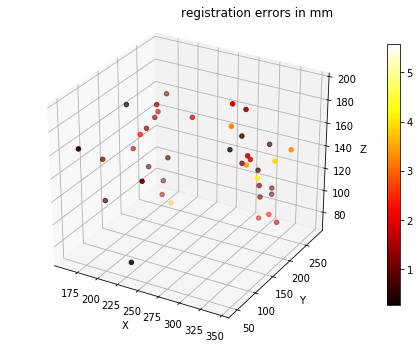Final alignment errors in millimeters, mean(std): 1.63(1.18), max: 5.57

Quantitative Evaluation II (Segmentation)¶

While the use of corresponding points to evaluate registration is the desired approach, it is often not applicable. In many cases there are only a few distinct points which can be localized in the two images, possibly too few to serve as a metric for evaluating the registration result across the whole region of interest.

An alternative approach is to use segmentation. In this approach, we independently segment the structures of interest in the two images. After registration we transfer the segmentation from one image to the other and compare the original and registration induced segmentations.

In :
# Transfer the segmentation via the estimated transformation.
# Nearest Neighbor interpolation so we don't introduce new labels.
fixed_image,
tx,
sitk.sitkNearestNeighbor,
0.0,

We have now replaced the task of evaluating registration with that of evaluating segmentation.

In :
# Often referred to as ground truth, but we prefer reference as the truth is never known.
# Segmentations before and after registration
segmentations = [moving_image_mask, transformed_labels == utilities.popi_lung_label]
In :
from enum import Enum

# Use enumerations to represent the various evaluation measures
class OverlapMeasures(Enum):
jaccard, dice, volume_similarity, false_negative, false_positive = range(5)

class SurfaceDistanceMeasures(Enum):
hausdorff_distance, mean_surface_distance, median_surface_distance, std_surface_distance, max_surface_distance = range(5)

# Empty numpy arrays to hold the results
overlap_results = np.zeros((len(segmentations),len(OverlapMeasures.__members__.items())))
surface_distance_results = np.zeros((len(segmentations),len(SurfaceDistanceMeasures.__members__.items())))

# Compute the evaluation criteria

# Note that for the overlap measures filter, because we are dealing with a single label we
# use the combined, all labels, evaluation measures without passing a specific label to the methods.
overlap_measures_filter = sitk.LabelOverlapMeasuresImageFilter()

hausdorff_distance_filter = sitk.HausdorffDistanceImageFilter()

# Use the absolute values of the distance map to compute the surface distances (distance map sign, outside or inside
# relationship, is irrelevant)
label = 1
reference_distance_map = sitk.Abs(sitk.SignedMaurerDistanceMap(reference_segmentation, squaredDistance=False))
reference_surface = sitk.LabelContour(reference_segmentation)

statistics_image_filter = sitk.StatisticsImageFilter()
# Get the number of pixels in the reference surface by counting all pixels that are 1.
statistics_image_filter.Execute(reference_surface)
num_reference_surface_pixels = int(statistics_image_filter.GetSum())

for i, seg in enumerate(segmentations):
# Overlap measures
overlap_measures_filter.Execute(reference_segmentation, seg)
overlap_results[i,OverlapMeasures.jaccard.value] = overlap_measures_filter.GetJaccardCoefficient()
overlap_results[i,OverlapMeasures.dice.value] = overlap_measures_filter.GetDiceCoefficient()
overlap_results[i,OverlapMeasures.volume_similarity.value] = overlap_measures_filter.GetVolumeSimilarity()
overlap_results[i,OverlapMeasures.false_negative.value] = overlap_measures_filter.GetFalseNegativeError()
overlap_results[i,OverlapMeasures.false_positive.value] = overlap_measures_filter.GetFalsePositiveError()
# Hausdorff distance
hausdorff_distance_filter.Execute(reference_segmentation, seg)
surface_distance_results[i,SurfaceDistanceMeasures.hausdorff_distance.value] = hausdorff_distance_filter.GetHausdorffDistance()
# Symmetric surface distance measures
segmented_distance_map = sitk.Abs(sitk.SignedMaurerDistanceMap(seg, squaredDistance=False))
segmented_surface = sitk.LabelContour(seg)

# Multiply the binary surface segmentations with the distance maps. The resulting distance
# maps contain non-zero values only on the surface (they can also contain zero on the surface)
seg2ref_distance_map = reference_distance_map*sitk.Cast(segmented_surface, sitk.sitkFloat32)
ref2seg_distance_map = segmented_distance_map*sitk.Cast(reference_surface, sitk.sitkFloat32)

# Get the number of pixels in the segmented surface by counting all pixels that are 1.
statistics_image_filter.Execute(segmented_surface)
num_segmented_surface_pixels = int(statistics_image_filter.GetSum())

# Get all non-zero distances and then add zero distances if required.
seg2ref_distance_map_arr = sitk.GetArrayViewFromImage(seg2ref_distance_map)
seg2ref_distances = list(seg2ref_distance_map_arr[seg2ref_distance_map_arr!=0])
seg2ref_distances = seg2ref_distances + \
list(np.zeros(num_segmented_surface_pixels - len(seg2ref_distances)))
ref2seg_distance_map_arr = sitk.GetArrayViewFromImage(ref2seg_distance_map)
ref2seg_distances = list(ref2seg_distance_map_arr[ref2seg_distance_map_arr!=0])
ref2seg_distances = ref2seg_distances + \
list(np.zeros(num_reference_surface_pixels - len(ref2seg_distances)))

all_surface_distances = seg2ref_distances + ref2seg_distances

surface_distance_results[i,SurfaceDistanceMeasures.mean_surface_distance.value] = np.mean(all_surface_distances)
surface_distance_results[i,SurfaceDistanceMeasures.median_surface_distance.value] = np.median(all_surface_distances)
surface_distance_results[i,SurfaceDistanceMeasures.std_surface_distance.value] = np.std(all_surface_distances)
surface_distance_results[i,SurfaceDistanceMeasures.max_surface_distance.value] = np.max(all_surface_distances)

import pandas as pd
from IPython.display import display, HTML

# Graft our results matrix into pandas data frames
overlap_results_df = pd.DataFrame(data=overlap_results, index=["before registration", "after registration"],
columns=[name for name, _ in OverlapMeasures.__members__.items()])
surface_distance_results_df = pd.DataFrame(data=surface_distance_results, index=["before registration", "after registration"],
columns=[name for name, _ in SurfaceDistanceMeasures.__members__.items()])

# Display the data as HTML tables and graphs
display(HTML(overlap_results_df.to_html(float_format=lambda x: '%.3f' % x)))
display(HTML(surface_distance_results_df.to_html(float_format=lambda x: '%.3f' % x)))
overlap_results_df.plot(kind='bar', rot=1).legend(bbox_to_anchor=(1.6,0.9))
surface_distance_results_df.plot(kind='bar', rot=1).legend(bbox_to_anchor=(1.6,0.9));
jaccard dice volume_similarity false_negative false_positive
before registration 0.882 0.937 0.065 0.031 0.092
after registration 0.965 0.982 -0.001 0.018 0.017
hausdorff_distance mean_surface_distance median_surface_distance std_surface_distance max_surface_distance
before registration 18.045 1.341 1.000 1.642 18.055
after registration 8.831 0.377 0.000 0.921 17.833